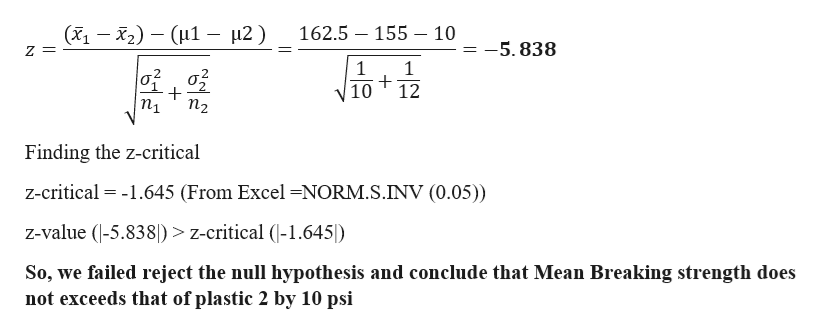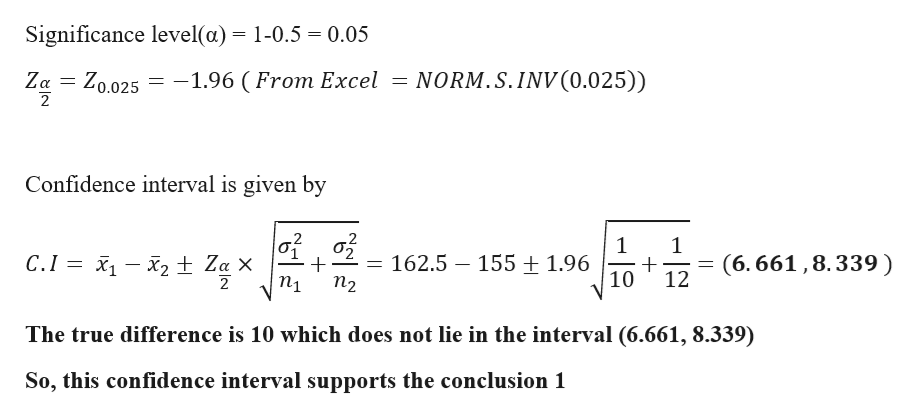# For the problems below, when asked to conduct the hypothesis test, please use the seven-stepprocess we have been using in class. Also, in step 7, it is very important to be clear in yourconclusion. There are three parts to this: evidence from your hypothesis test, the decision to rejector fail to reject the null hypothesis, and the conclusion in the context of the problem. For examplereferring to the first example we did in Chapter 10, we stated the following:"-1.03 is not less than -1.96, nor is it greater than +1.96, so we fail to reject Ho: u1 -H2 = 0, andconclude that there is no difference in mean yield strength between the two types of roof bolts."(Here, the evidence is-1.03 is not less than -1.96, nor is it greater than +1.96The decision isWe fail to reject Ho: H1-2 0The conclusion in the context of the problem isWe conclude that there is no difference in mean yield strength between the two types ofroof bolts)Two types of plastic are suitable for an electronics component manufacturer to use, and thebreaking strength of this plastic is important. It is known that o1 02 = 1.0 psi. From a randomsample of size n1= 10 and n2 = 12, you obtain 162.5 psi and 2 155.0 psi. The company willnot adopt plastic 1 unless its mean breaking strength exceeds that of plastic 2 by at least 10 psi. Usea 0.05 for all parts of the problem.(a) Use the seven-step hypothesis test that we have been using in class to determine if the companyshould use plastic 1. Do not use the P-Value(b) Determine the proper confidence interval or bound for this situation, and calculate it. Describewhy this confidence interval/bound supports your conclusion from part (a)(c) Suppose that the true difference in means is 12.0 psi. Determine the probability of a Type II1error 1. Identify the parameter of interest. Describe it in the context of thesituation.2. Determine null value and state null hypothesis.3. State the appropriate alternative hypothesis.4. Give the formula for the computed value of the test statistic.5. State the rejection region for the selected level of significance, a.6. Compute sample quantities (if necessary), substitute into formula for thetest statistic value, and compute that value (z in this case).7. Decide whether or not the null hypothesis should be rejected and state theconclusion in the context of the original problem. (Either "reject" or "fail toreject the null hypothesis; never "accept" the null hypothesis. Next,explain what this means in terms of your specific situation, e.g., "concludethat the mean sulfur content is greater than 1.50%.")

Question
12 views

THIS NOT GRADED ASSIGIMENT , IT IS AN OLD EXAM QUESTION.

check_circle

Step 1

Given data

Step 2

a)

Formulation of Hypothesis

Null Hypothesis: - Mean Breaking strength exceeds that of plastic 2 by at least 10 psi

i.e. H0: µ1- µ2  ≥ 10

Alternative Hypothesis: - Mean Breaking strength does not exceeds that of plastic 2 by 10 psi

i.e. H1: µ1 - µ2 < 10

Test statistichelp_outlineImage Transcriptionclose(-2)(H1 H2) 162.5 155 - 10 5.838 Z = 1 1 12 V10 п1 Finding the z-critical z-critical 1.645 (From Excel NORM.S.INV (0.05)) z-value (-5.838) z-critical (-1.645) So, we failed reject the null hypothesis and conclude that Mean Breaking strength does not exceeds that of plastic 2 by 10 psi fullscreen
Step 3

b)

Finding the 95 % confidence interval...help_outlineImage TranscriptioncloseSignificance level(a) = 1-0.5 = 0.05 NORM.S.INV(0.025)) Ζα Ζ0.025 -1.96 ( From Excel Confidence interval is given by 1 162.5 155 ± 1.96 10 1 (6. 661,8.339) 12 C.I -2 + Za X 2 n2 пу The true difference is 10 which does not lie in the interval (6.661, 8.339) So, this confidence interval supports the conclusion 1 fullscreen

### Want to see the full answer?

See Solution

#### Want to see this answer and more?

Solutions are written by subject experts who are available 24/7. Questions are typically answered within 1 hour.*

See Solution
*Response times may vary by subject and question.
Tagged in
MathStatistics

### Hypothesis Testing